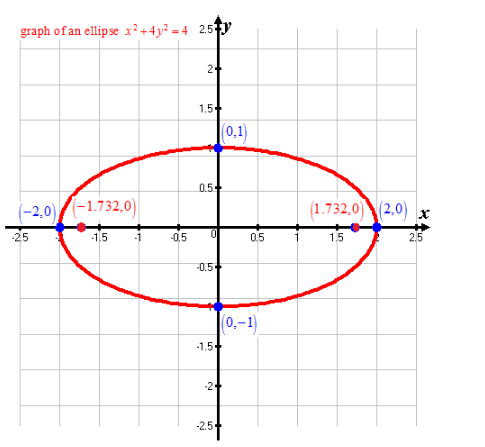# To find the foci of the ellipse x 2 + 4 y 2 = 4 and sketch its graph### Single Variable Calculus: Concepts...

4th Edition
James Stewart
Publisher: Cengage Learning
ISBN: 9781337687805### Single Variable Calculus: Concepts...

4th Edition
James Stewart
Publisher: Cengage Learning
ISBN: 9781337687805

#### Solutions

Chapter B, Problem 50E
To determine

## To calculate: To find the foci of the ellipse x2+4y2=4 and sketch its graph

Expert Solution

The foci of ellipse are (3,0) and (3,0)

### Explanation of Solution

Given information: Equation of ellipse is x2+4y2=4

Formula Used:

Standard equation of ellipse is

x2a2+y2b2=1 ,

where c2=a2b2

Foci of above ellipse is (±c,0)

Calculation:

Equation of ellipse is given as

x2+4y2=4

Rewrite the equation as follows:

x24+4y24=44x24+y21=1x222+y212=1

On comparing the equation with standard equation of ellipse,

a=2

b=1

Value of c is calculated as follows:

c2=a2b2c2=2212c2=41c2=3c=±3

Thus, foci of ellipse are (3,0) and (3,0)

On sketching the ellipse:Conclusion:

Hence, foci of ellipse are (3,0) and (3,0)

### Have a homework question?

Subscribe to bartleby learn! Ask subject matter experts 30 homework questions each month. Plus, you’ll have access to millions of step-by-step textbook answers!